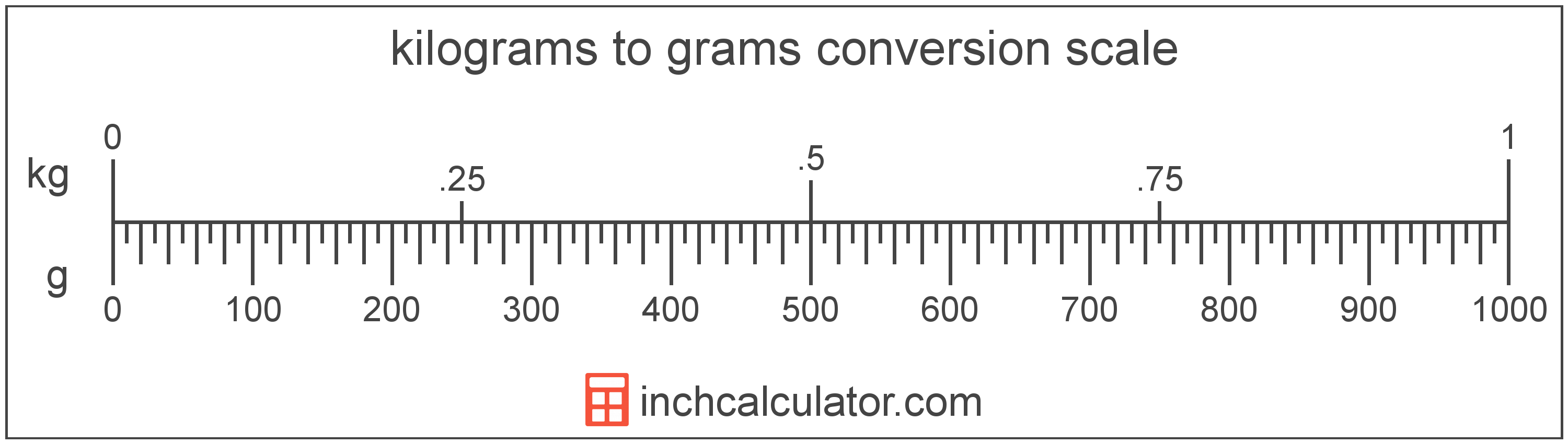# Kilograms to Grams Converter

Enter the weight in kilograms below to get the value converted to grams.

Results in Grams:1 kg = 1,000 g

Do you want to convert grams to kilograms?

## How to Convert Kilograms to Grams

To convert a measurement in kilograms to a measurement in grams, multiply the weight by the following conversion ratio: 1,000 grams/kilogram.

Since one kilogram is equal to 1,000 grams, you can use this simple formula to convert:

grams = kilograms × 1,000

The weight in grams is equal to the weight in kilograms multiplied by 1,000.

For example, here's how to convert 5 kilograms to grams using the formula above.
grams = (5 kg × 1,000) = 5,000 g### How Many Grams Are in a Kilogram?

There are 1,000 grams in a kilogram, which is why we use this value in the formula above.

1 kg = 1,000 g

## What Is a Kilogram?

One kilogram is equal to 1,000 grams, 2.204623 pounds, or 1/1,000 of a metric ton.

The formal definition of the kilogram changed in 2019. One kilogram was previously equal to the mass of the platinum-iridium bar, known as the International Prototype of the Kilogram, which was stored in Sèvres, France.

The 2019 SI brochure now defines the kilogram using the Planck constant, and it is defined using the meter and second. It is equal to the mass of 1,000 cubic centimeters, or milliliters, of water.

The kilogram, or kilogramme, is the SI base unit for weight and is also a multiple of the gram. In the metric system, "kilo" is the prefix for thousands, or 103. Kilograms can be abbreviated as kg; for example, 1 kilogram can be written as 1 kg.

## What Is a Gram?

A gram is a unit of mass equal to 1/1,000 of a kilogram or 0.035274 ounces, and is equivalent to the mass of one cubic centimeter, or one milliliter, of water.

The gram, or gramme, is an SI unit of weight in the metric system. Grams can be abbreviated as g; for example, 1 gram can be written as 1 g.

## Kilogram to Gram Conversion Table

Table showing various kilogram measurements converted to grams.
Kilograms Grams
0.001 kg 1 g
0.002 kg 2 g
0.003 kg 3 g
0.004 kg 4 g
0.005 kg 5 g
0.006 kg 6 g
0.007 kg 7 g
0.008 kg 8 g
0.009 kg 9 g
0.01 kg 10 g
0.02 kg 20 g
0.03 kg 30 g
0.04 kg 40 g
0.05 kg 50 g
0.06 kg 60 g
0.07 kg 70 g
0.08 kg 80 g
0.09 kg 90 g
0.1 kg 100 g
0.2 kg 200 g
0.3 kg 300 g
0.4 kg 400 g
0.5 kg 500 g
0.6 kg 600 g
0.7 kg 700 g
0.8 kg 800 g
0.9 kg 900 g
1 kg 1,000 g

## References

1. International Bureau of Weights and Measures, The International System of Units, 9th Edition, 2019, https://www.bipm.org/documents/20126/41483022/SI-Brochure-9-EN.pdf